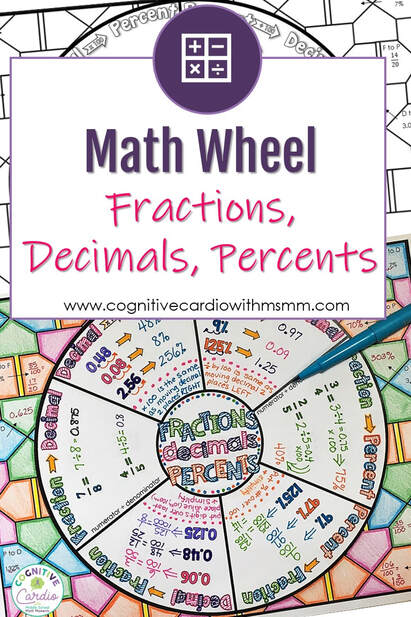# Fraction, Decimal, Percent Math WheelI’m really liking the math wheel idea, so I created a new math wheel for fraction, decimal, percent conversion notes:-)​How to use this resource (this information is also in the free download):
Around the outside of the wheel are the different conversion headings – you can use the wheel to introduce the conversions, filling in just the ones you are covering each day. Or, you can use it to review all the conversions at once. In either case, the wheel can be kept in students’ notebooks as a reference/study tool.

1) I like to begin with decimal to percent and percent to decimal. In the arrows in these sections, you’ll see x 100 and ÷ 100. It think it’s important that students understand that these are the operations being used for these conversions before giving them a shortcut, so I let them use calculators to complete the examples. Once the examples are complete, I ask the students to look for the pattern – what happens to the decimal point in each of these cases?
We decide on the “shortcut” rules together and then write them at the bottom of those sections.

2) The fraction to percent and fraction to decimal sections have the rules written already, so the examples just need to be completed.
I always relate fraction to percent to students grades. By the time we get to this topic during the year, students have been figuring out their grades for months (I never write their percentages on their assessments – they need to calculate
them). They know how to find their percentage if their quiz grade was 6/8 or their test was 48/52. However, sometimes they need a reminder that this official fraction to percent “rule” is the same thing they’ve been doing for months! I have them
write a  little reminder in that section – “just like test grades!”

3)  For percent to fraction, students need to remember that percent means “out of 100,” so the percent number will always go over 100. Then they must reduce.

4)  I find that decimal to fraction is sometimes tricky for students. When they have trouble, I ask them to read the decimal number according to place value (“How do you say this number, using tenths, hundredths, or thousandths, etc.?”). Once
they speak it, they know how to write the fraction – 0.27 is 27 hundredths, which is 27/100. After completing the examples, we discuss the idea that the denominator will be whatever the last decimal place is (10, 100, 1000, etc.) and the numerator will be the digits in the decimal number. We write this rule as simply as possible.

5)  Students then complete the 10 problems around the page. Above each number is the conversion to complete (F to P, P to D, etc.) They can then color the rest of the wheel background.

I had a great time coloring my answer key! These could make a fun decoration as well:-)

I hope you can use it!!

Interested in more math wheels? Check out these free problem solving wheels!

## EllieWelcome to Cognitive Cardio Math! I’m Ellie, a wife, mom, grandma, and dog ‘mom,’ and I’ve spent just about my whole life in school! With nearly 30 years in education, I’ve taught:

• All subject areas in 4th and 5th grades
• Math, ELA, and science in 6th grade (middle school)

I’ve been creating resources for teachers since 2012 and have worked in the elearning industry for about five years as well!

If you’re looking for ideas and resources to help you teach math (and a little ELA), I can help you out!